## Scaling Images

By default, images (such as JPEG, PNG, BMP, SVG) that are placed in panels are not scaled. This section demonstrates the four different scaling options available.

Here’s a multi-panel figure with four panels, to contain the images. In each case, we’ll use the copy of the R logo contained in the multipanelfigure package. This is a PNG file, 800 pixels wide and 700 pixels high, with a resolution of 300 dots per inch (DPI). That gives it a native size of 2.67" by 2.33".

``````library(multipanelfigure)
figure <- multi_panel_figure(
width = c(1, 5),
height = c(1, 1, 4),
unit = "inches")
r_logo <- system.file("extdata/Rlogo.png", package = "multipanelfigure")``````

By default, the image is not rescaled, which means that only the central part of the image is displayed.

``(figure %<>% fill_panel(r_logo))``
``## Setting row to 1``
``## Setting column to 1``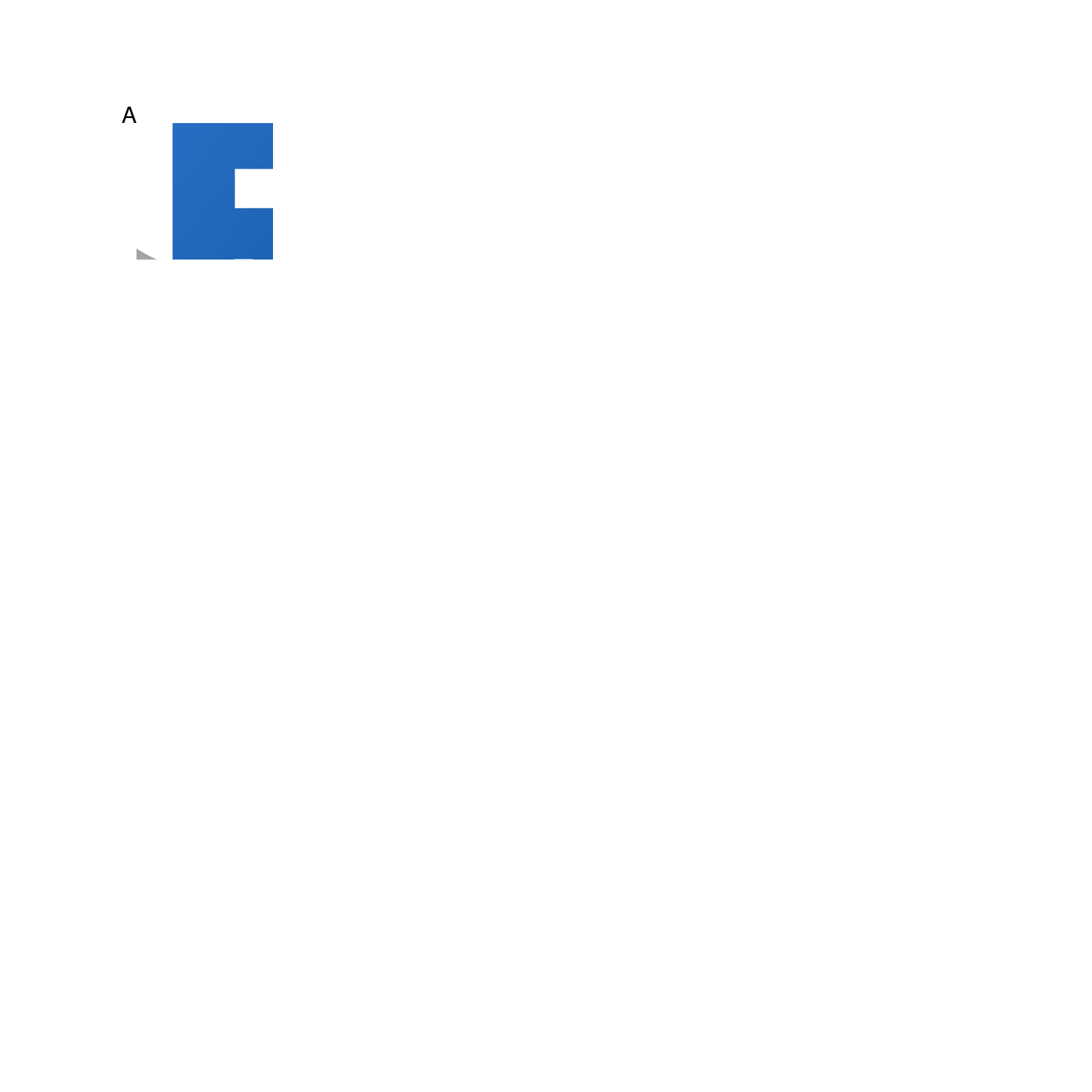By setting `scaling = "stretch"`, all the image is displayed, fitting the panel exactly, but the height-to-width ratio had been altered, distorting the image.

``(figure %<>% fill_panel(r_logo, scaling = "stretch"))``
``## Setting row to 1``
``## Setting column to 2``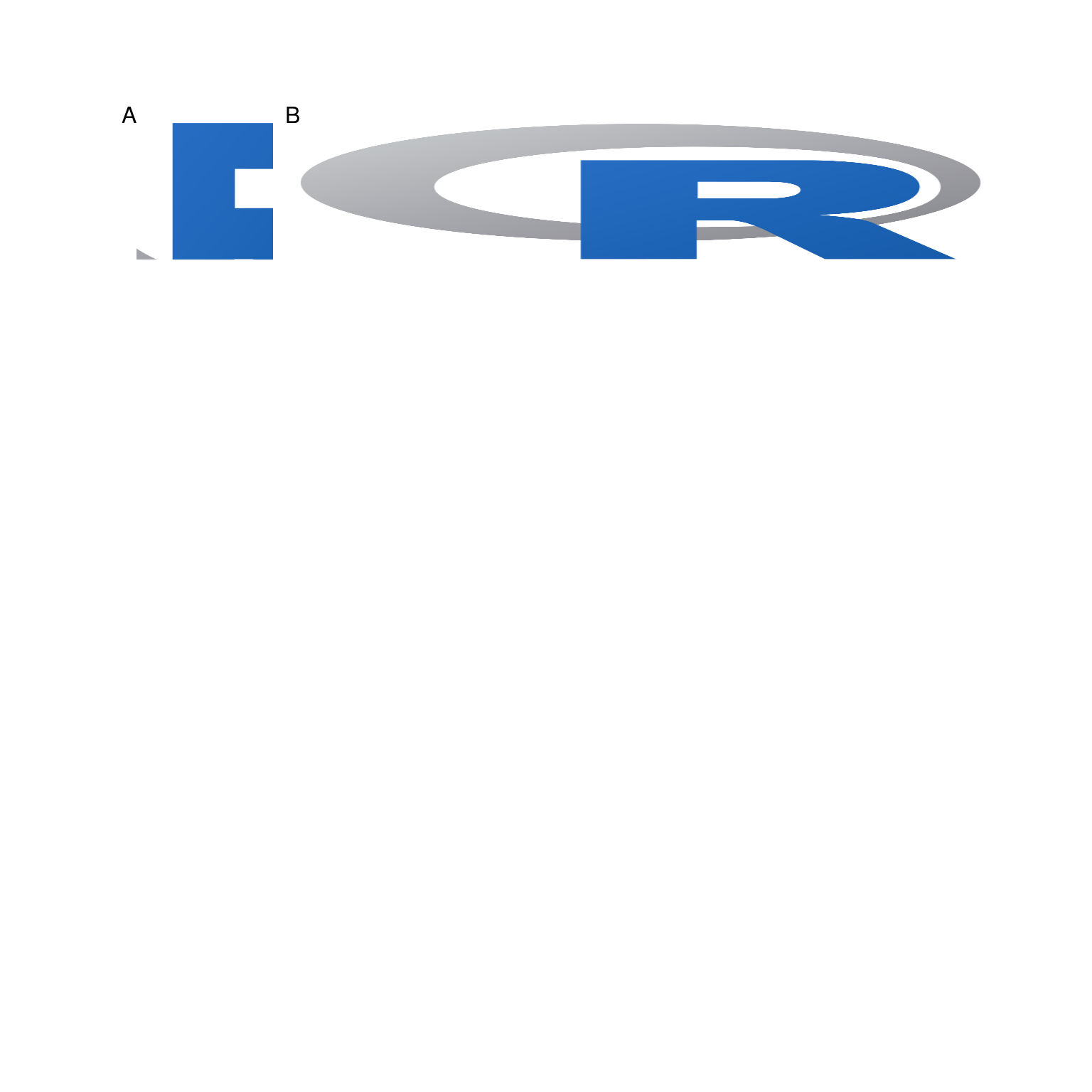Setting `scaling = "fit"` means that the image is shrunked or grown so that it just fits inside the panel without the height-to-width ratio being distorted.

``(figure %<>% fill_panel(r_logo, scaling = "fit"))``
``## Setting row to 2``
``## Setting column to 1``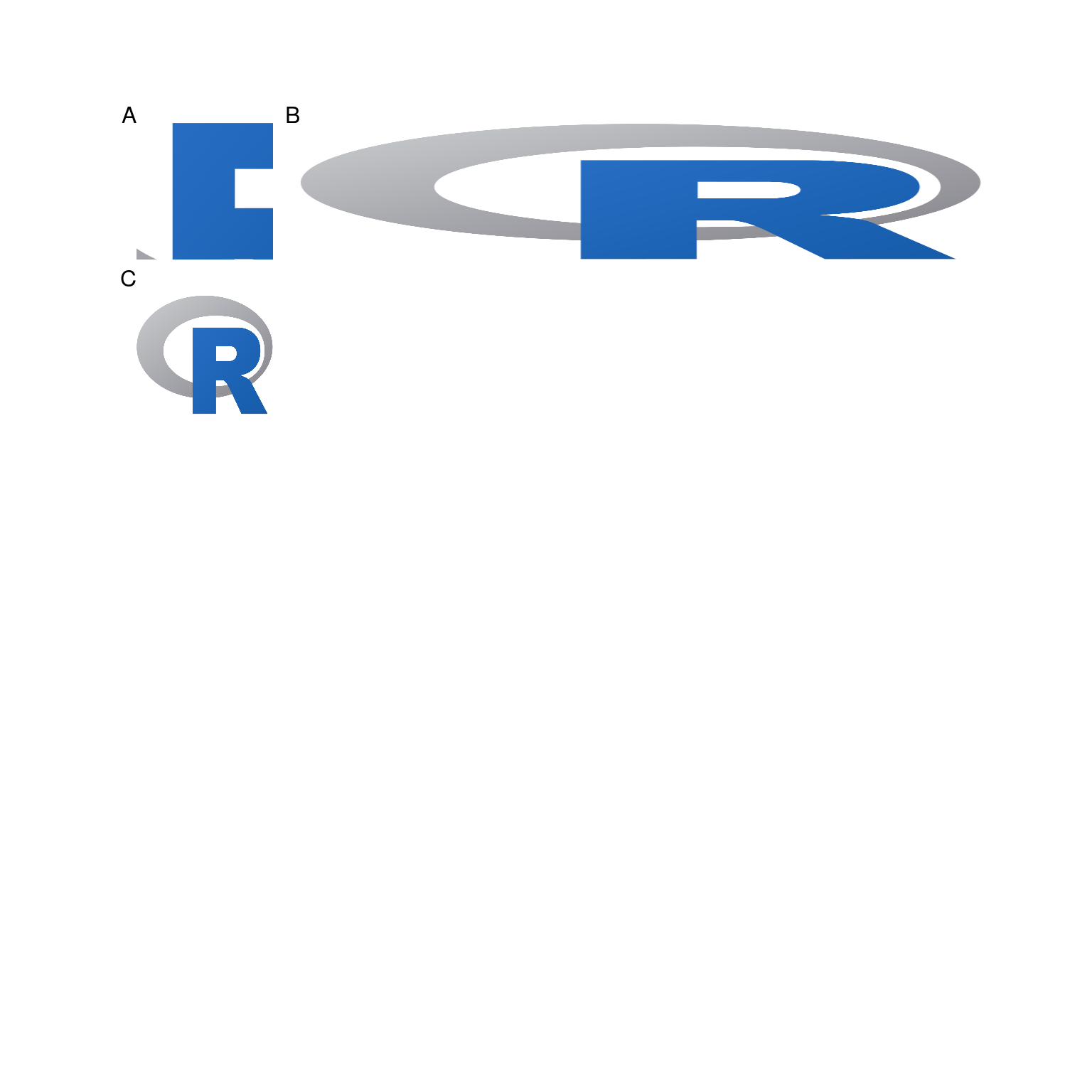Setting `scaling = "shrink"`, works the same way as `scaling = "fit"` when the image is larger than the panel.

``(figure %<>% fill_panel(r_logo, scaling = "shrink", row = 3, column = 1))``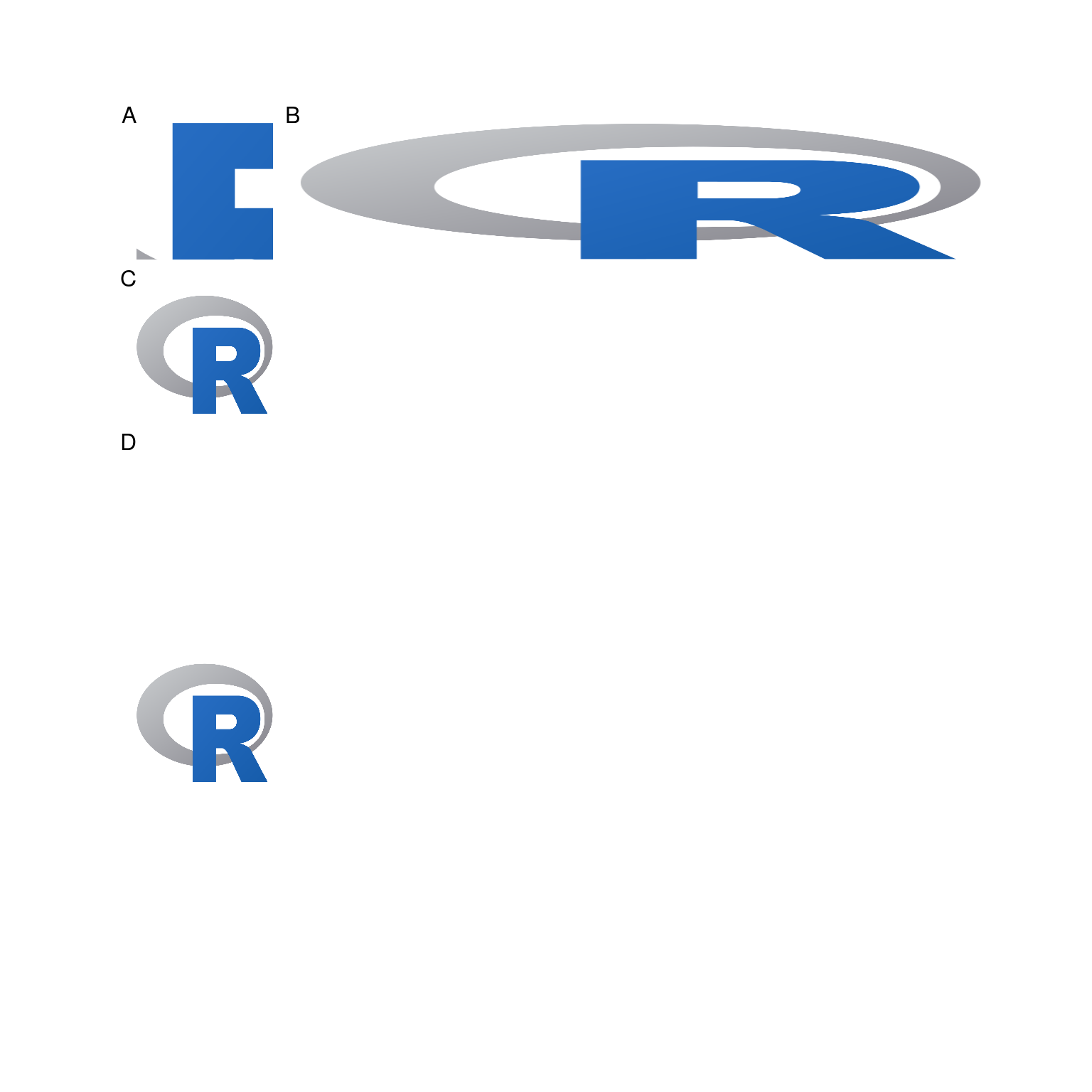If the image is smaller than the panel, `scaling = "shrink"` works like `scaling = "none"`.

``(figure %<>% fill_panel(r_logo, scaling = "shrink", row = 2:3, column = 2))``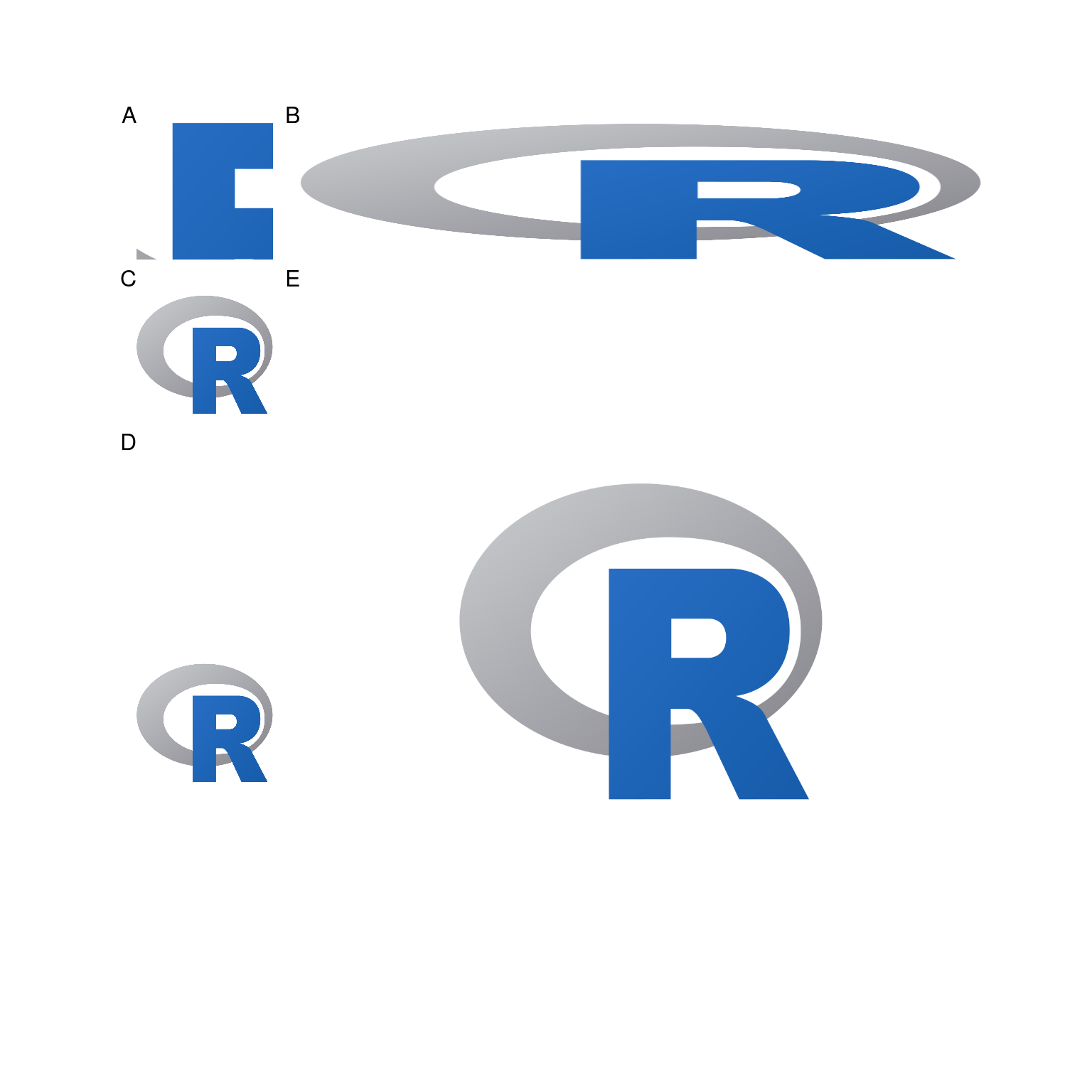## Clipping Plots and Images

By default, plots and images are clipped so that they don’t overrun the panel that they were put in. (See, for example, Panel A in the previous plot.) To demonstrate the effect of clipping, let’s use a 3 by 3 multi-panel figure, and fill all but the centre panel.

``````library(grid)
figure2 <- multi_panel_figure(
width = rep.int(1.25, 3),
height = rep.int(1.25, 3),
unit = "inches")
rhino <- system.file("extdata/rhino.jpg", package = "multipanelfigure")
for(position in list(c(1, 1), c(1, 2), c(1, 3), c(2, 1), c(2, 3), c(3, 1), c(3, 2), c(3, 3)))
{
figure2 %<>%
fill_panel(
rectGrob(gp = gpar(fill = "#91A45E")),
row = position,
column = position)
}
figure2``````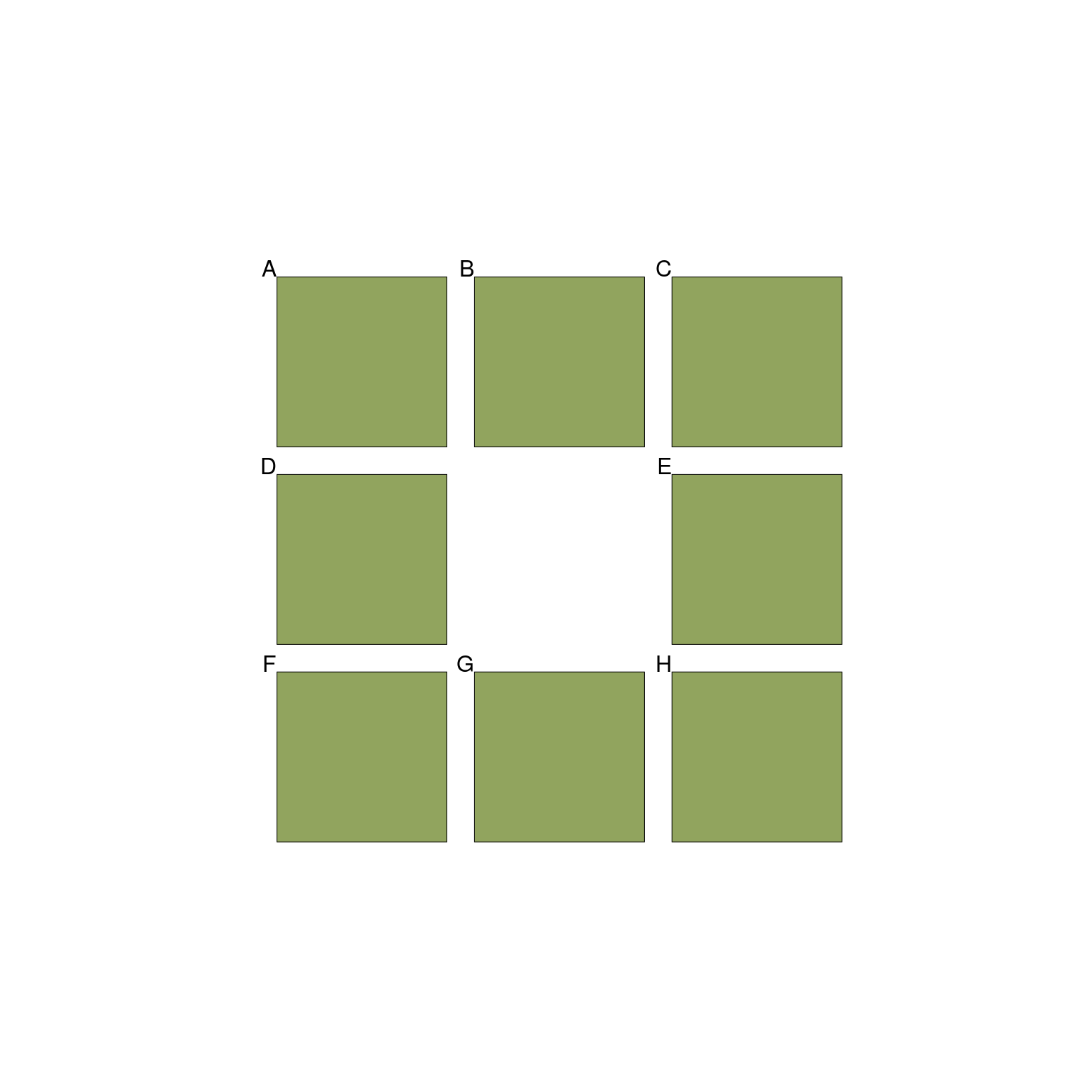When we try to display the rhino, only the central part of the image is shown, since there is no rescaling, and the panel isn’t big enough.

``(figure2 %>% fill_panel(rhino))``
``## Setting row to 2``
``## Setting column to 2``